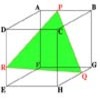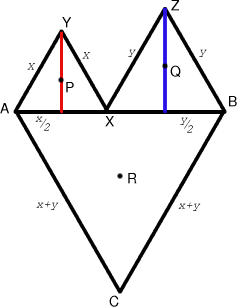#### You may also like### Cut Cube

Find the shape and symmetries of the two pieces of this cut cube.### Cushion Ball

The shortest path between any two points on a snooker table is the straight line between them but what if the ball must bounce off one wall, or 2 walls, or 3 walls?### Plane to See

P is the midpoint of an edge of a cube and Q divides another edge in the ratio 1 to 4. Find the ratio of the volumes of the two pieces of the cube cut by a plane through PQ and a vertex.

# Napoleon's Hat

##### Age 16 to 18 Challenge Level:This is Sue Liu's solution proving that the triangle $PQR$ is equilateral whatever the position of the point $X$. Many congratulations Sue on all your excellent work.

Let $AX = x$ and $XB = y$ where we know that $AX + XB = AB$ (constant). Let the points $P, Q$ and $R$ be the centres (centroids) of the triangles $\Delta AXY, \Delta XZB$ and $\Delta ABC$ respectively. We use the fact that the medians of a triangle intersect at the centroid and this point divides the medians in the ratio one third to two thirds. If we set the point $A$ as the origin, then the points $P$, $Q$ and $R$, being the centroids of the equilateral triangles $AXY$, $XZB$ and $ABC$, have coordinates

$P = ({1\over 2}x, {\sqrt 3\over 6}x),$

$Q = (x + {1\over 2}y, {\sqrt 3\over 6}y)$, and

$R = ({1\over 2}(x + y), {-\sqrt 3\over 6}(x +y)).$

We now show that the lengths $PQ$, $QR$ and $RP$ are equal. \eqalign{ PQ^2 &= (x + {1\over 2}y - {1\over 2}x)^2 + ({\sqrt 3\over 6}y - {\sqrt 3\over 6}x)^2 \cr &= {x^2 + 2xy + y^2\over 4} + {y^2 - 2xy + x^2 \over 12} \cr &= {x^2 +xy +y^2 \over 3}.} \eqalign { QR^2 &= ({1\over 2}(x + y) - (x + {1\over 2}y)^2 + (-{\sqrt 3\over 6}(x + y) - {\sqrt 3\over 6}y)^2 \cr &= {1\over 4}x^2 + {1\over 12}(x^2 + 4xy + 4y^2) \cr &= {x^2 +xy +y^2 \over 3}.} \eqalign { RP^2 &= ({1\over 2}(x + y) - {1\over 2}x)^2 + (-{\sqrt3 \over 6}(x + y) - {\sqrt 3\over 6}x)^2 \cr &= {1\over 4}y^2 + {1\over 12}(4x^2 + 4xy + y^2) \cr &= {x^2 +xy +y^2 \over 3}.} As $$PQ = QR = RP = \sqrt {{x^2 + xy + y^2 \over 3}}$$ for any $x$ it follows that $\Delta PQR$ is equilateral whatever the position of $X$.